Updated date:

# Physics Homework Questions: Examples of Average Velocity/Speed Problems With Step-by-Step SolutionsAll photos in hub, source: mariexotoni

## Motion Along a Straight Line: Average Velocity and Average Speed Problems With Solutions

Average velocity and average speed will fall under the Motion Along A Straight Line topic/ chapter in your textbook. I have done some of the problems, and the list of problems/solutions will continue to grow. Each problem will walk you through every step so you know how to obtain a solution.

Questions taken from Fundamentals of Physics, Halliday, the 9th Edition, extended.

Problems include: Chapter 2: 1, 3, 4, 6, 7

*If you came here for a specific problem, press Ctrl+F and type in some of the words in the problem to take you where you need to be.

## Problem Level: Easy

During a hard sneeze, your eyes might shut for 0.50 s. If you are driving a car at 90 km/h during such a sneeze, how far does the care move during that time?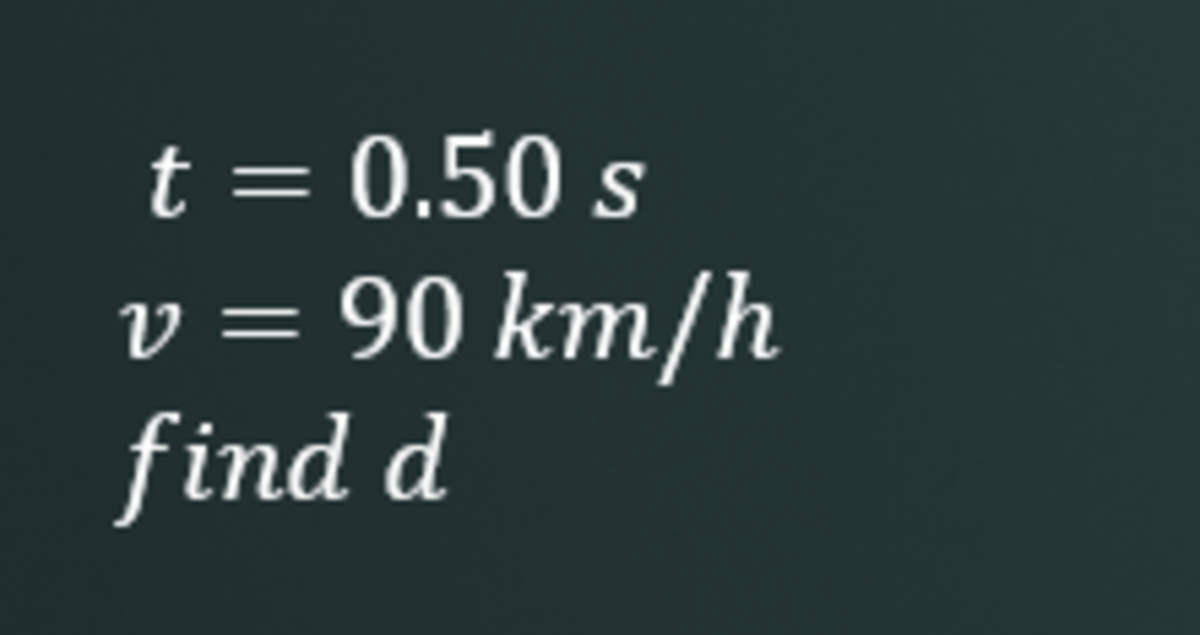1. List Your Givens

First things first, list all your given information so you can see what information you have. This will help you to plan out your next move. Just looking at the givens, you can easily see which equation you will be using to solve this problem.

2. Convert Where Necessary

You might have noticed that one of your givens isn't in standard units. To make this problem easier, convert your velocity to standard units which will be in meters per second.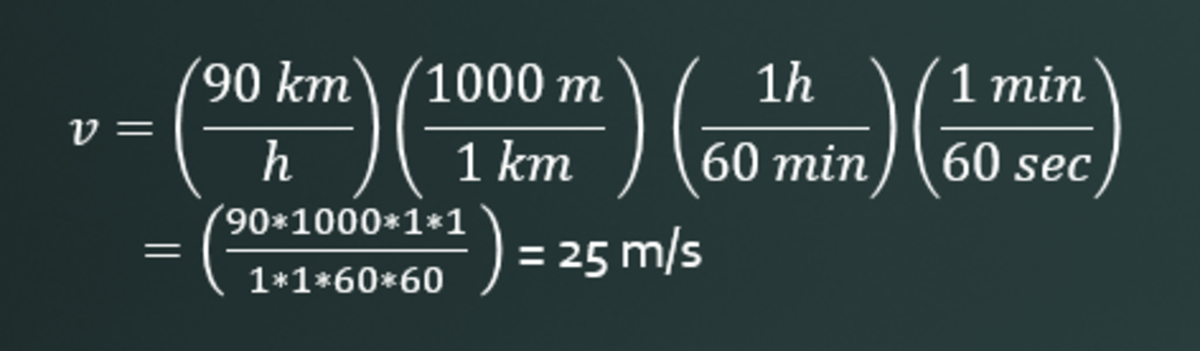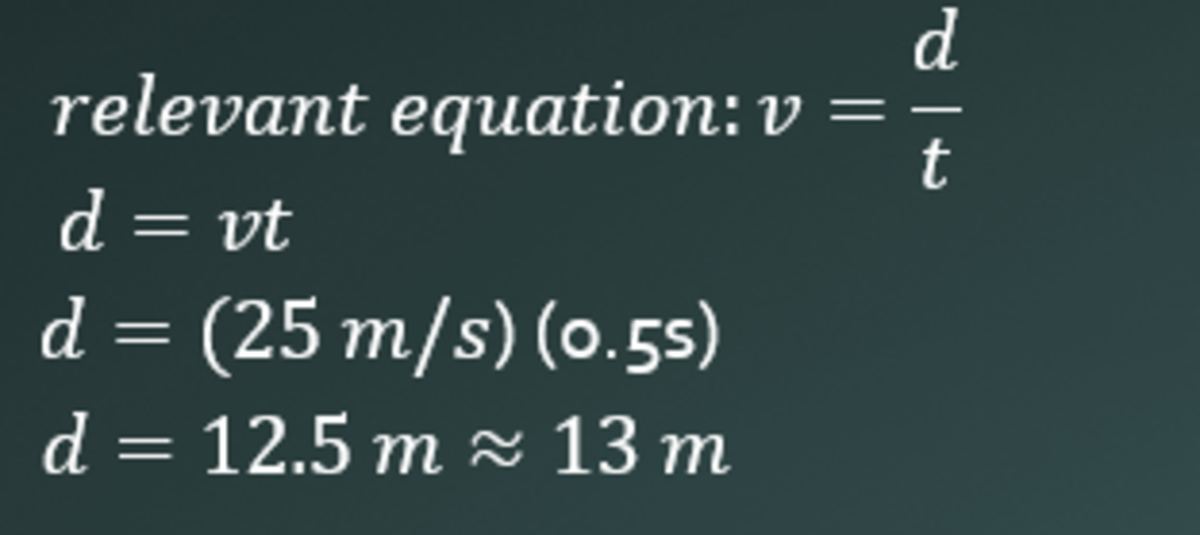3. Find Relevant Equation and Solve

For this problem, it's pretty obvious we are going to use the velocity equation. Remember, however, that you are solving for the distance, not the velocity. So, solve the equation for the distance. Although this really isn't necessary, you want to make a habit out of doing this. This will come in handy when the problems become more complex. Once you solve for the distance, plug in your information and solve.

Answer: 13 m

## Problem Level: Easy

An automobile travels on a straight road for 40 km at 30 km/h. It then continues in the same direction for another 40 km at 60 km/h. (a) What is the average velocity of the car during the full 80 km trip? (b) What is the average speed?vroom

1. Draw a Picture

Draw a picture for most of the physics problems you do is the best advice I can probably give you. Each segment in this picture represents 40 km.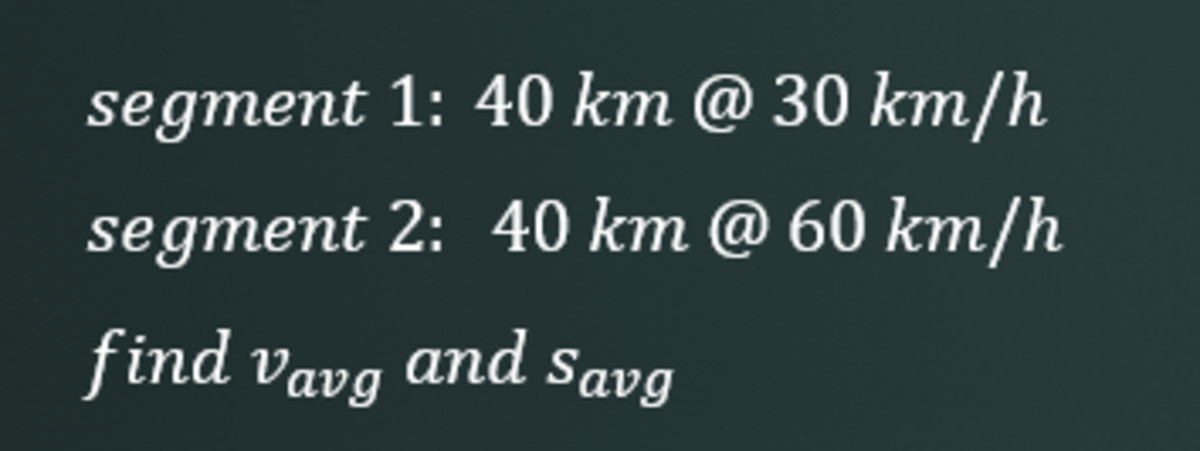2. List Your Givens

List your givens and what you have to find.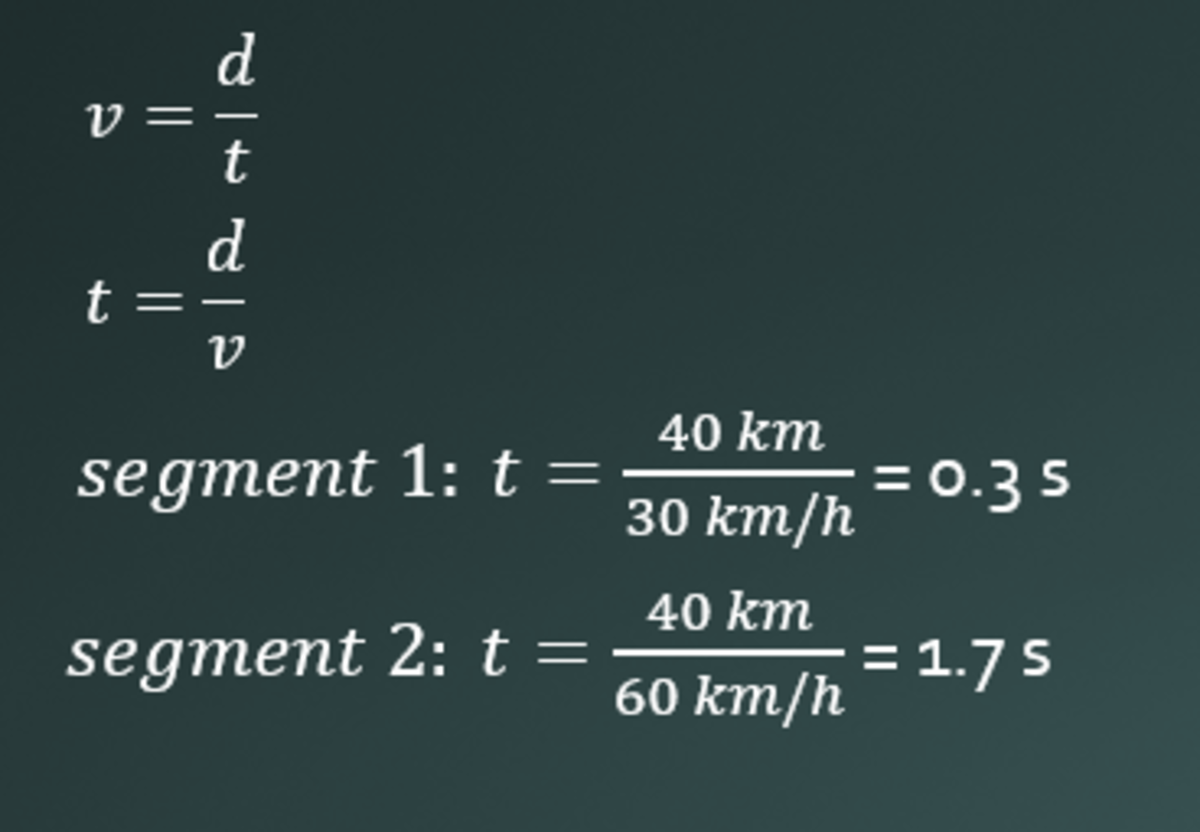3. Find Relevant Equations and Find the Times of Each Segment

Determine that the best equation to use for this problem is the velocity equation. Your first instinct may be to add both of the velocities and divide that number by 2 to get your average. However, since we aren't given the times of each segment, we can't just assume that they take the same amount of time. If each segment took an hour, for instance, you can add the velocities and divide by 2. Not in this case, however.

Take your velocity equation and solve for time. Plug in your values to find the times for each of the segments.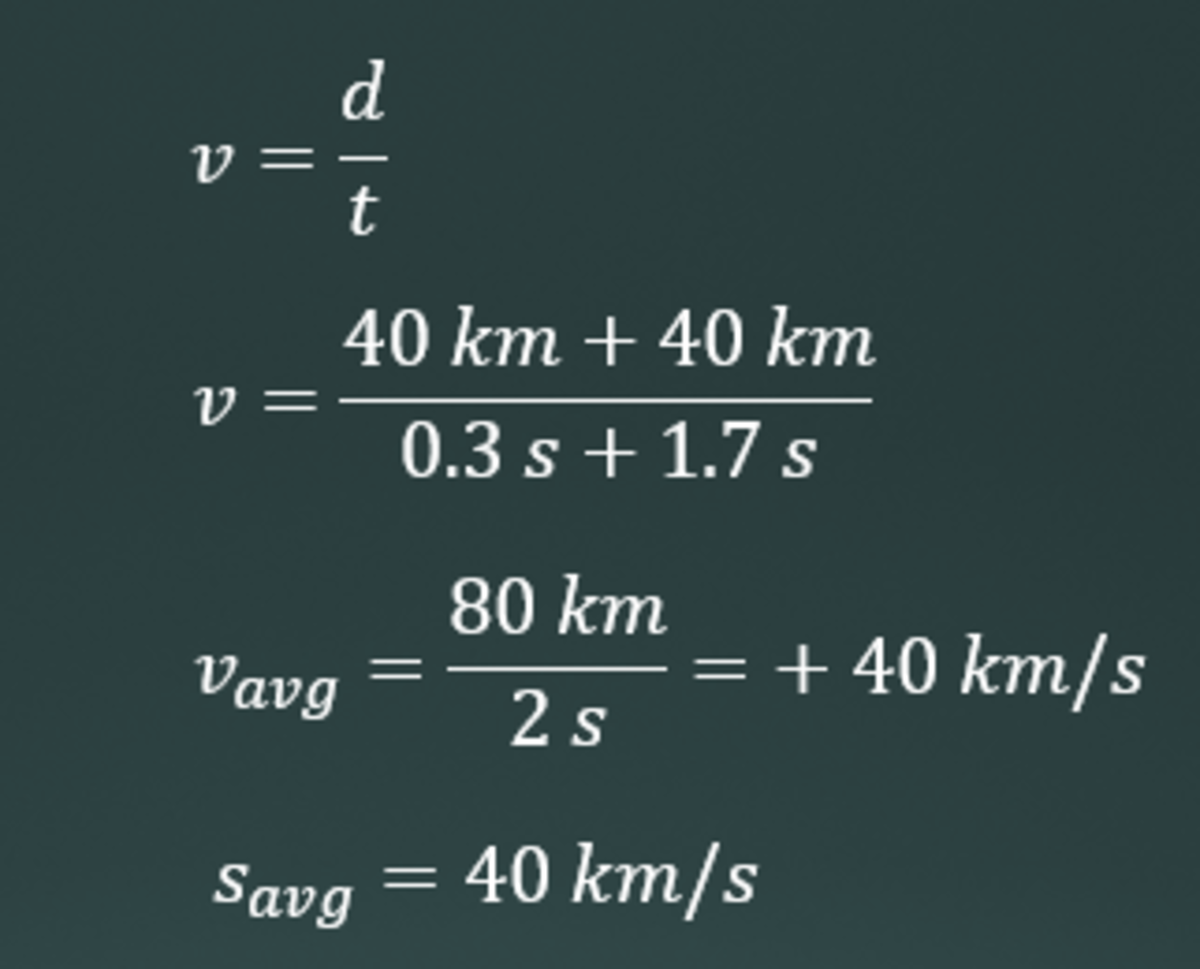4. Answer the Original Question: What's the Average Velocity?

To find the average velocity, we must find the total distance traveled and divide it by the change in time (which is the times totaled together). Then you solve.

In this case, the average velocity happens to be the same as the average speed. This isn't always the case, however. Remember that the speed is not a vector quantity, so the direction is irrelevant.

Answer: 40 km/s

## Problem Level: Easy

A car travels up a hill at a constant speed of 40 km/h and returns down the hill at a constant speed of 60 km/h. Calculate the average speed for the round trip.1. Draw Your Picture (Hopefully Better Than Mine)

Mine's pretty pathetic. But draw your picture so you can visualize what's going on. Each segment, in this case, represents some unknown distance you'll call x.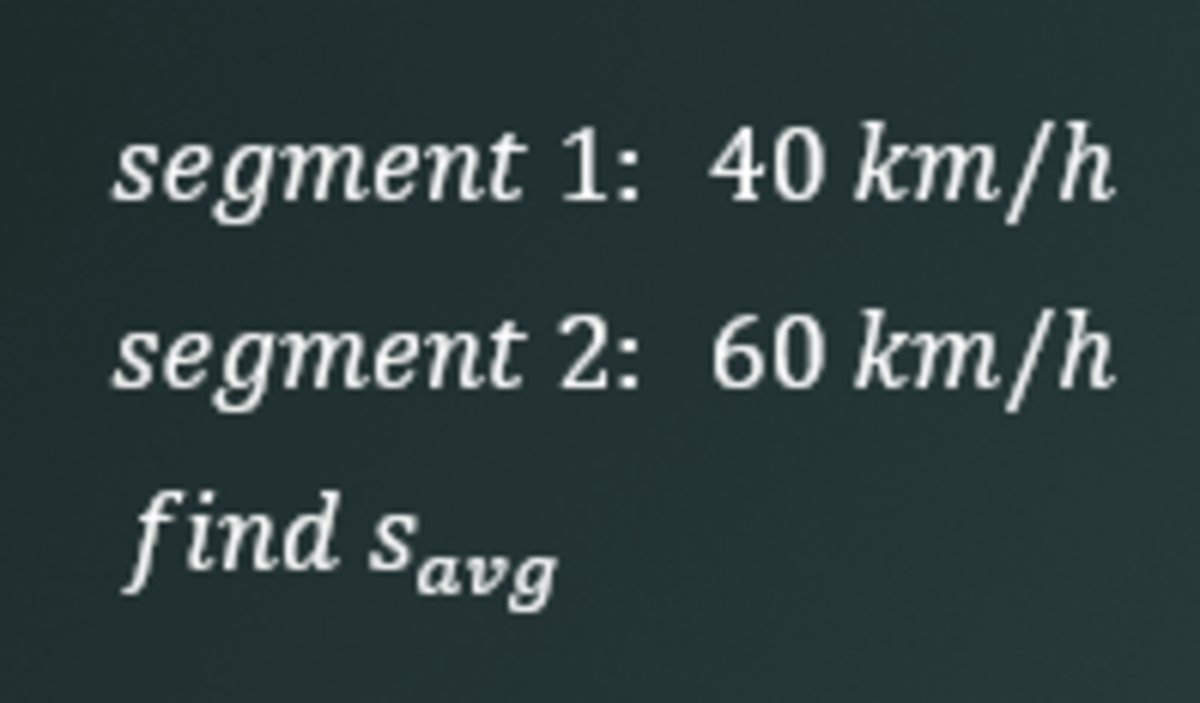2. List All Your Given Information

List all your given information—it doesn't have to look like mine or be set-up like mine.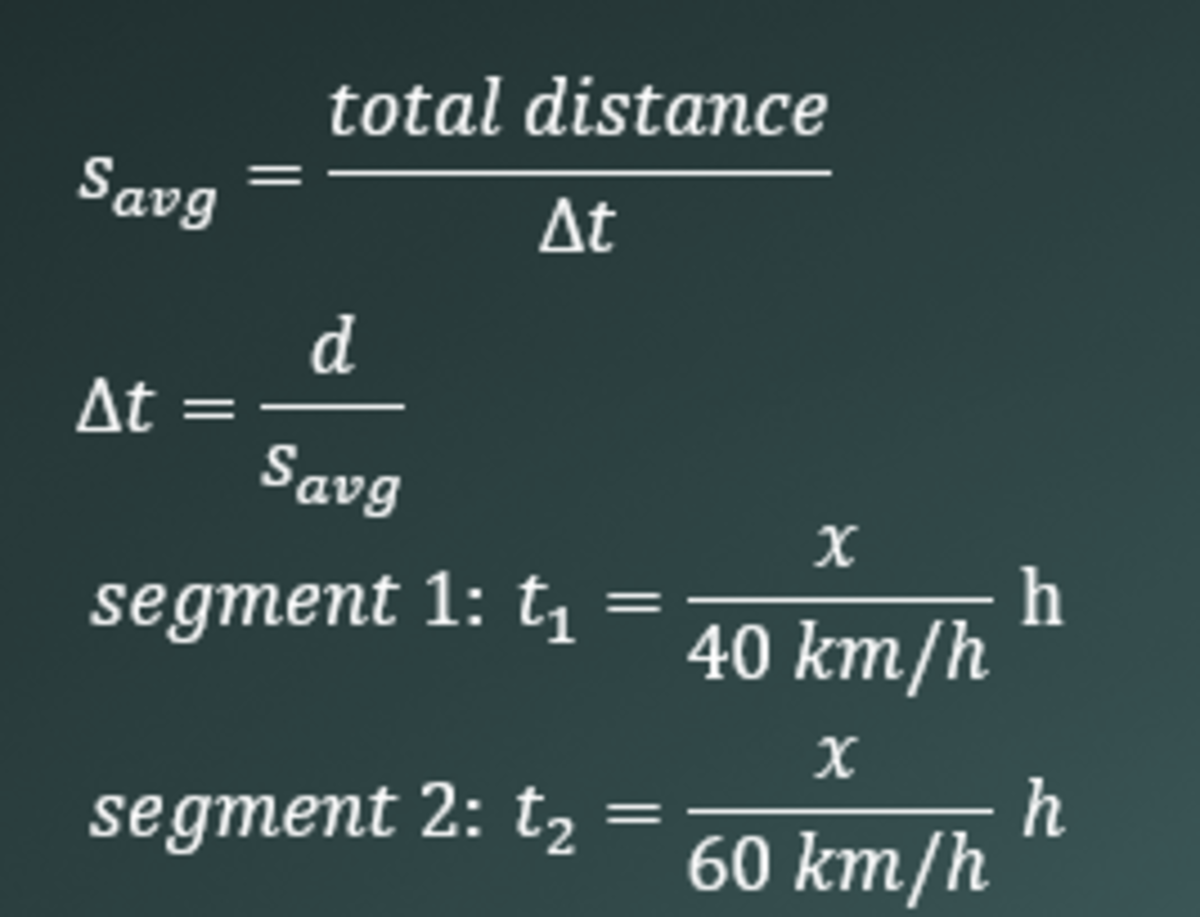3. Figure out Your Relevant Equations

We discussed earlier that your distance is some unknown value x. You also don't know your time, but it can easily be determined. Use your average speed equation and solve for the time. You will solve for the time for each segment. Plug in your velocities and d = x. You will not have some specific number. You will have x in your answer, but that's okay!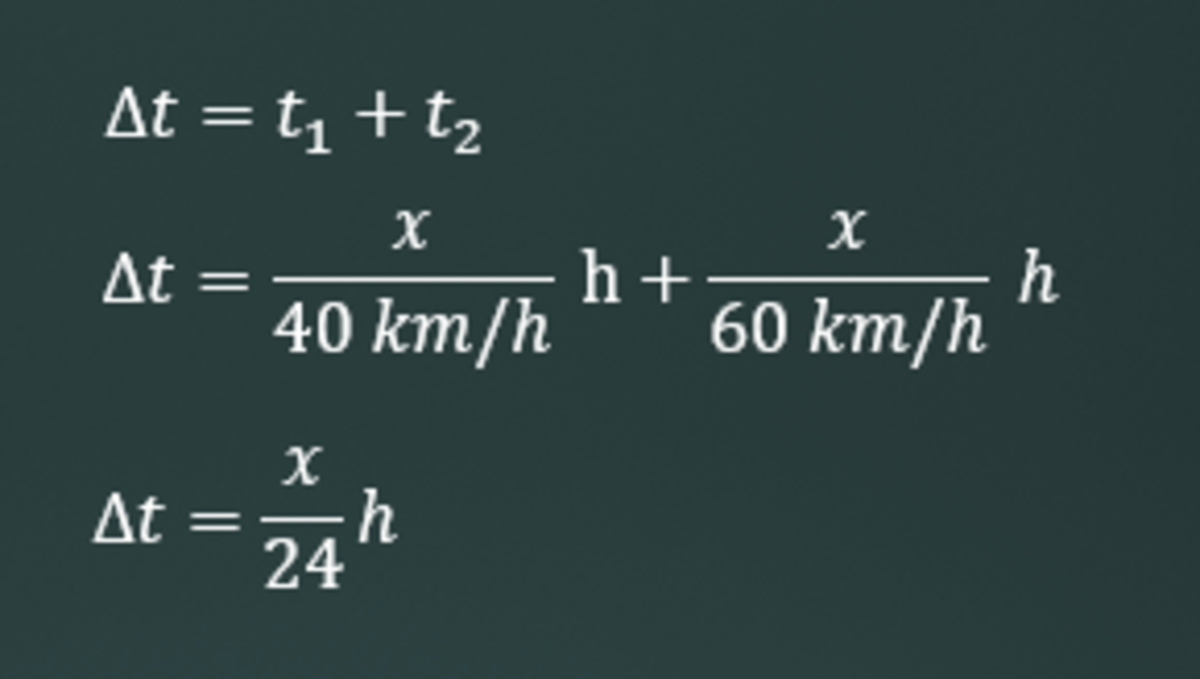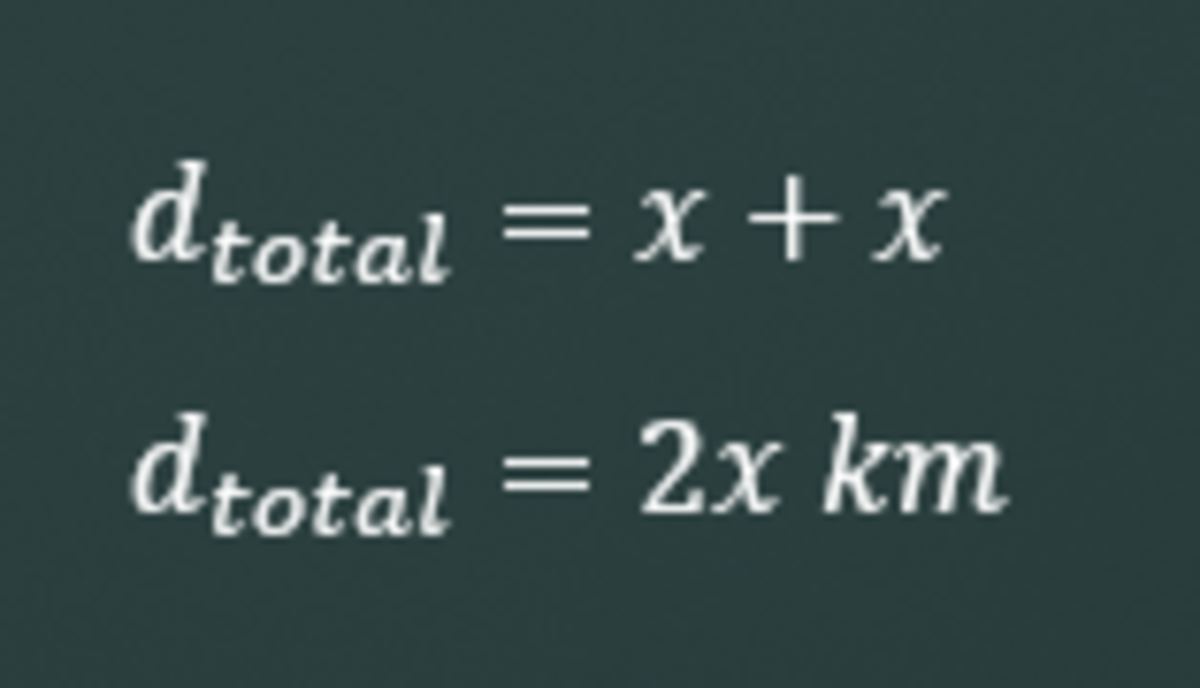4. Solve for Your Change in Time

Add the times of the two segments you just obtained. That is your change in time or the time it takes for the automobile to travel.

5. Solve for Your Total Distance

You have two unknown distances that you have assumed are the same length. Simply add your two unknown distances together to find the sum.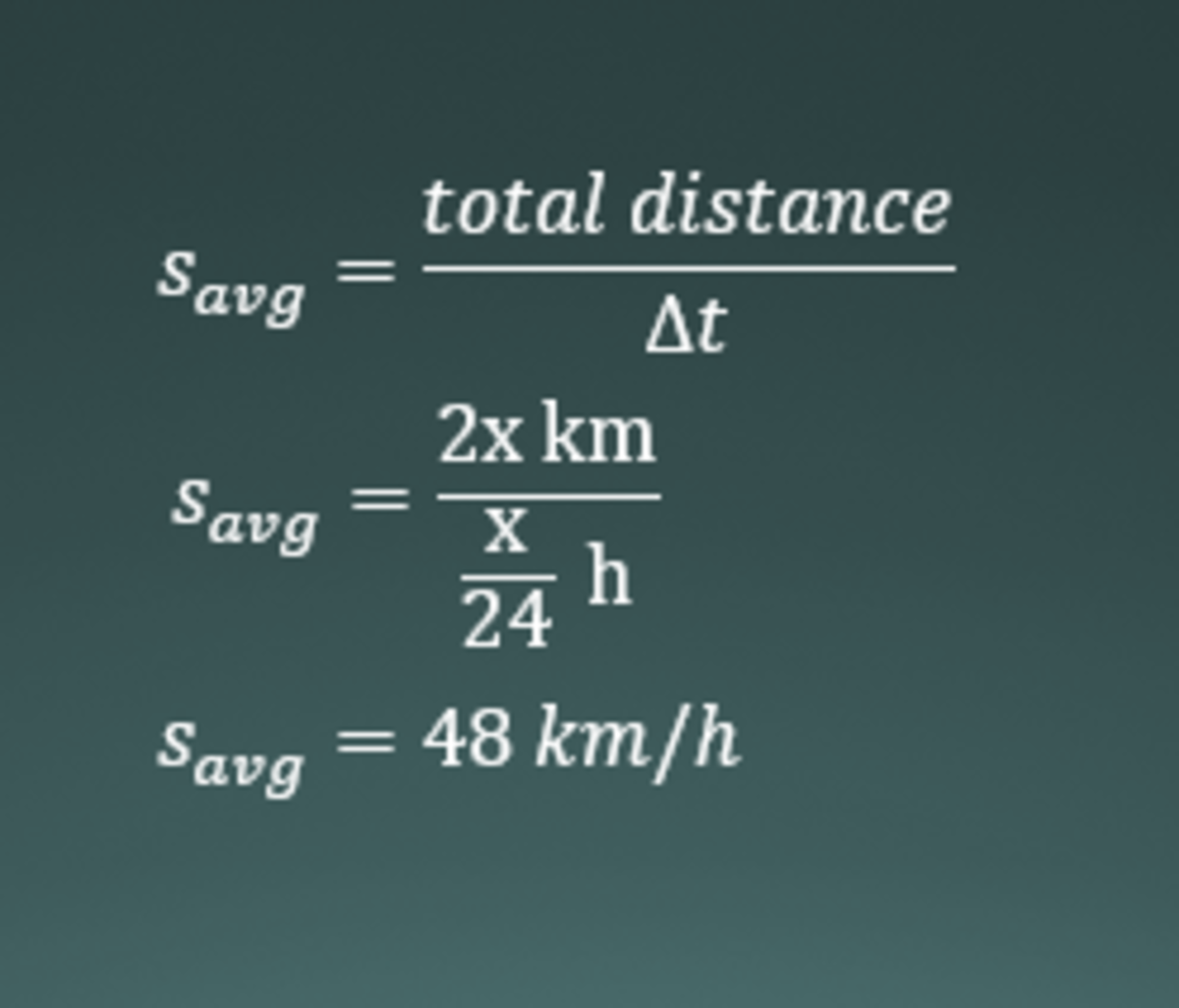6. Remember You're Solving for the Average Speed

You now have the change in time and the total distance. Plug this into your average speed equation and you will obtain the answer. (Remember: Keep, Change, Flip)

Answer: 48 km/h

## Problem Level: Easy

The 1992 world speed record for a bike (human powered vehicle) was set by Chris Huber (typo when I did the problem, thought it was Hubber), and his time through measured 200 m stretch was a sizzling 6.509 seconds, at which he commented, "Cogito erggoZoom!" Sam Whittingam beat Huber's record in 2001 by 19.0 km/h. what was Sam's time the 200 m?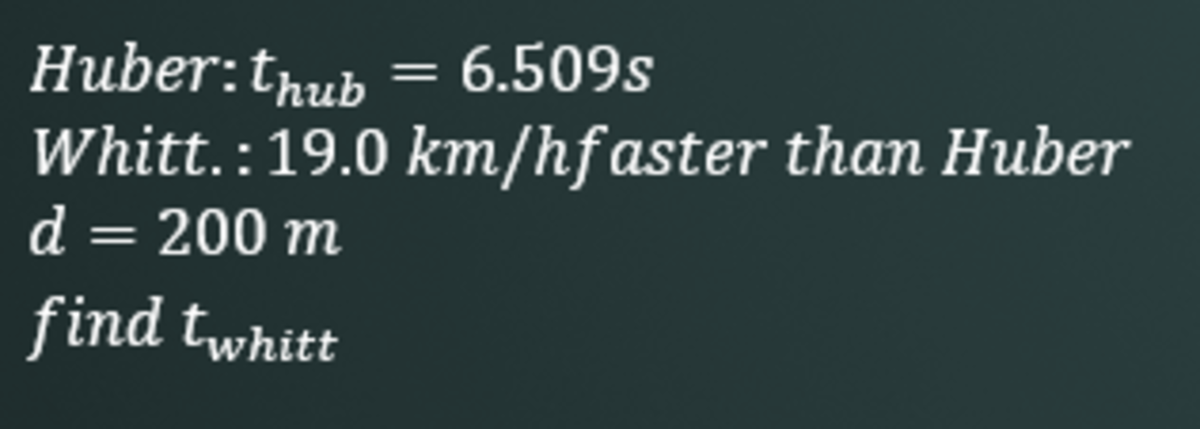1. List Your Given Information

Try to understand what the problem is telling you and what it's asking you to do.

2. Convert to Standard Units

Convert to standard units to make this problem easier to solve.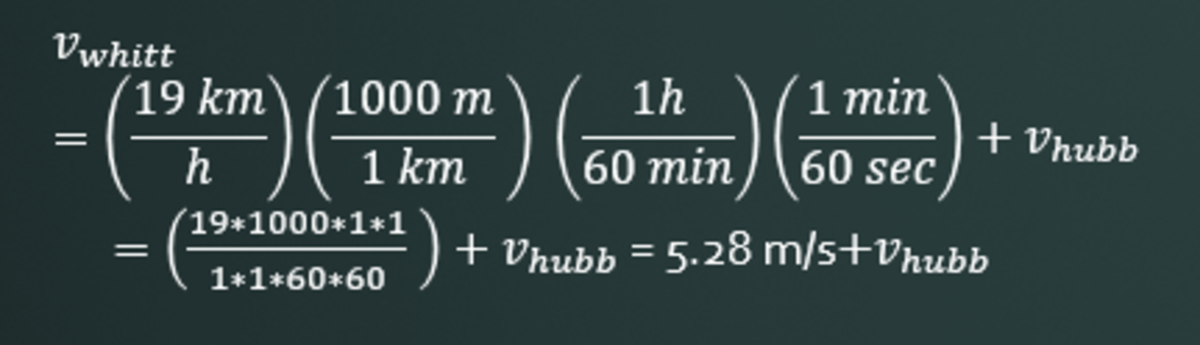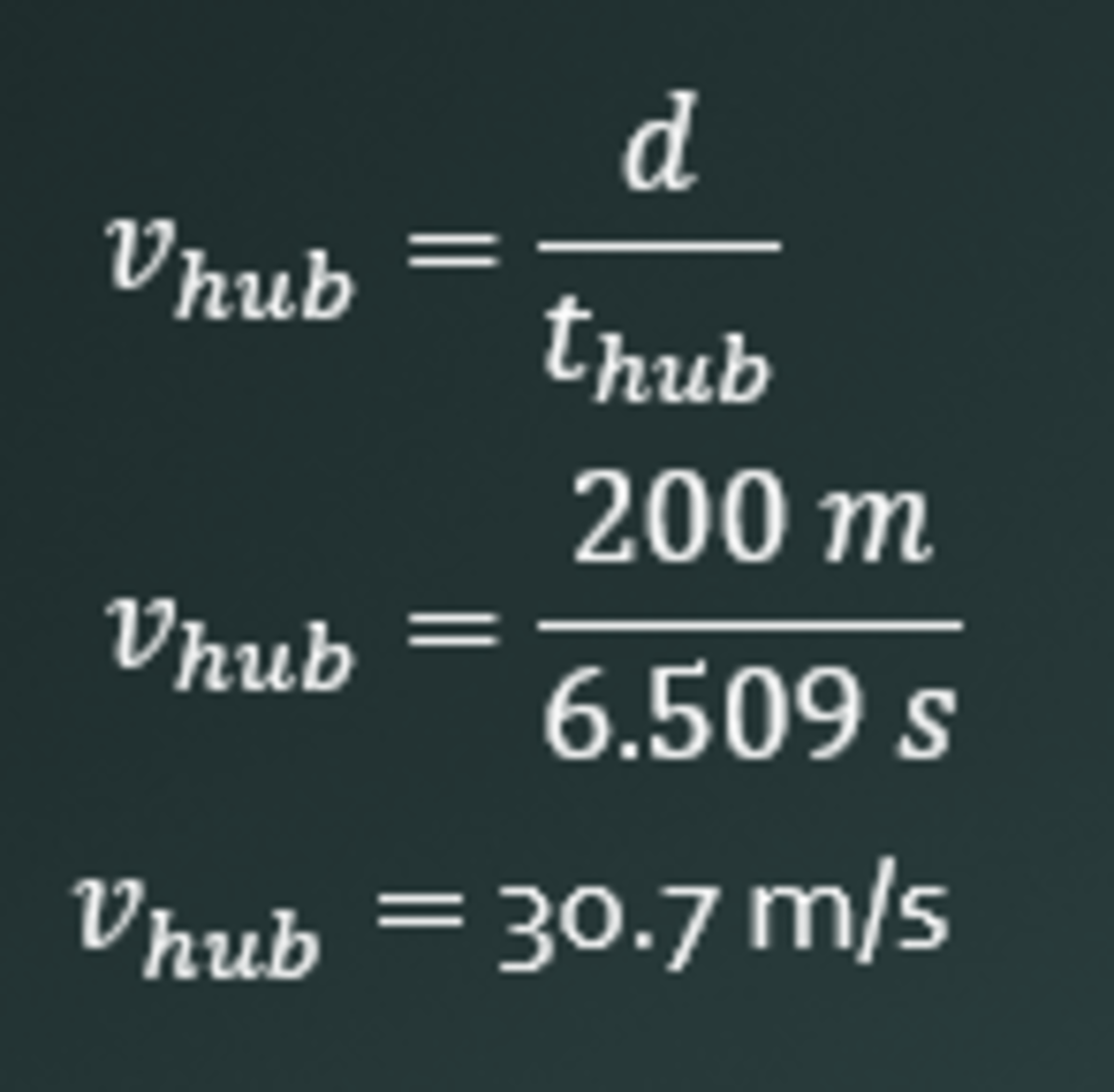3. Find the Velocity of Huber

You are going to be playing with and comparing velocities in this problem. You must solve Huber's velocity, so we can find Whitt.'s.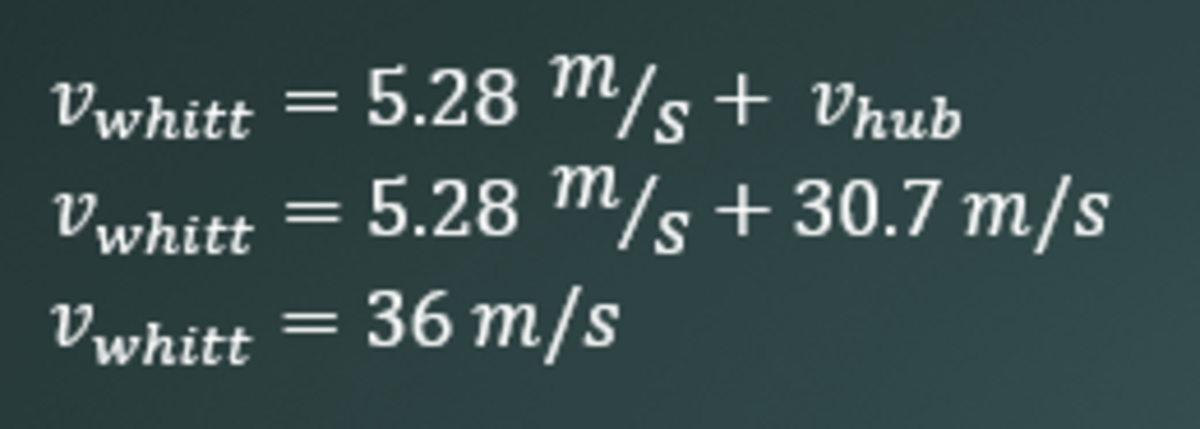4. Find the Velocity of Whitt

Since you know the Huber's velocity, you can now use that information, make an equation and solve for Whitt's velocity.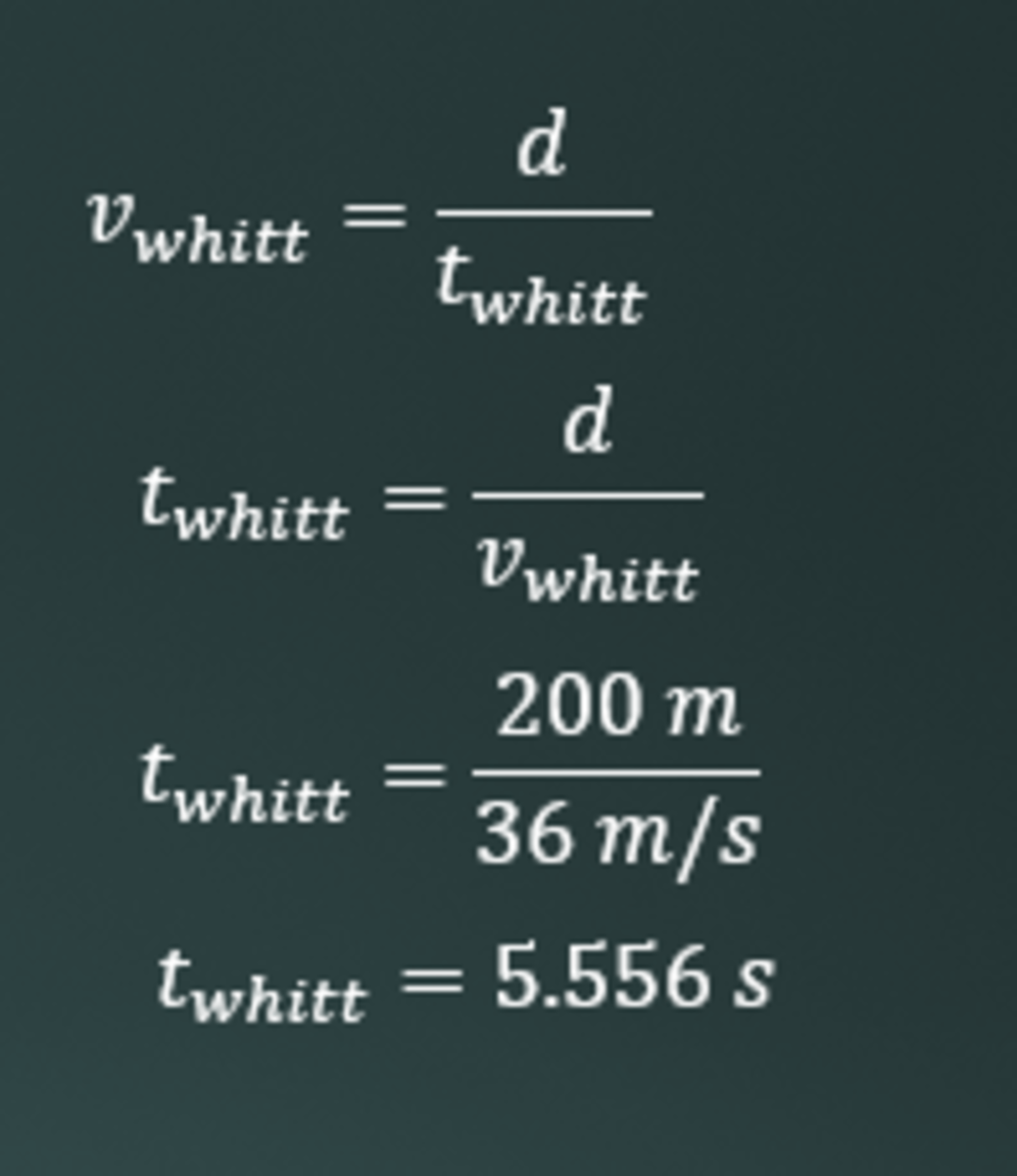5. You're Not Finished Yet! Remember What You're Looking For!

You don't want Whitt's velocity- you want his time. Easily, you can find the time using Whitt's velocity and the distance of the course.

Answer: 5.556 s

## Problem Level: Medium

Two trains, each having a speed of 30 km/h, are headed at each other on the same straight track. A bird that can fly at 60 km/h flies off the front of one of the train when they are 60 km apart and heads directly back to the first train, the bird flies directly back to the first train, and so on.What is the total distance the bird travels before the trains collide?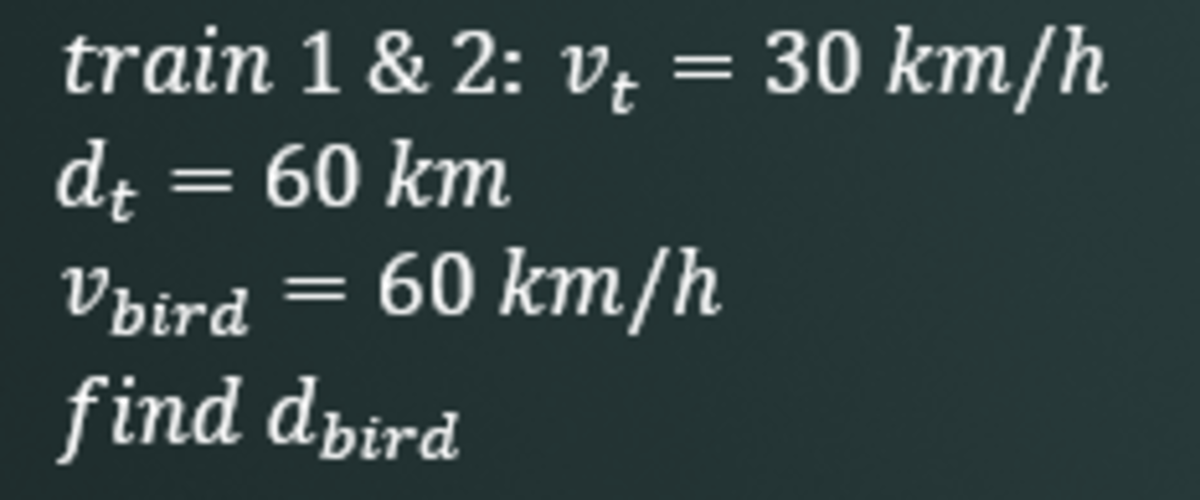1. List All Your Given Information

List it all. This problem is a little wordy, so you may have to read this a few more times more than you would with other problems. That's perfectly okay. I read this more than half a dozen times.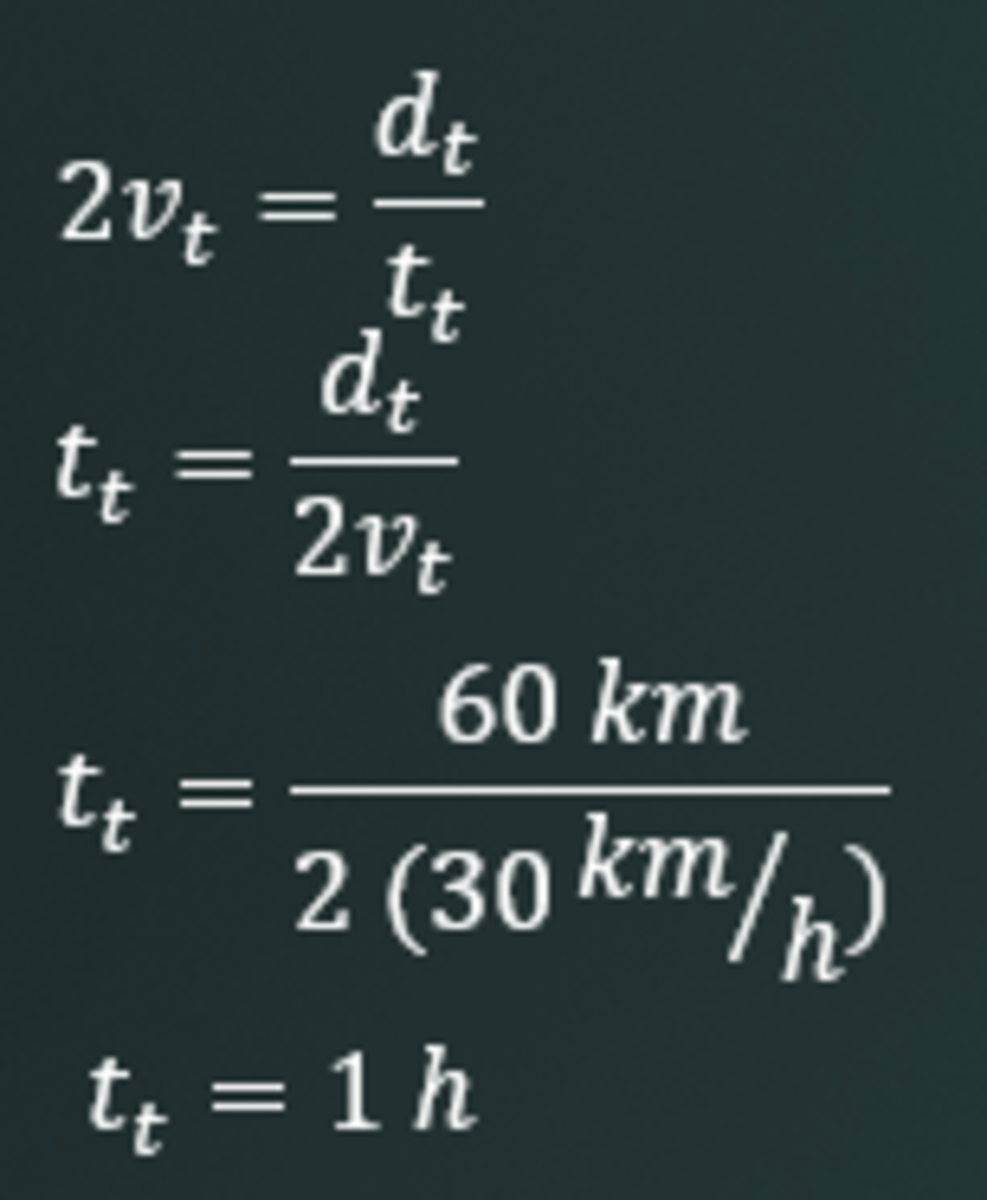2. Find the Time It Takes for the Two Trains to Collide

Since the trains are on the same track, you have to consider both of their velocities. Since they're the same, just double the velocity in your usual velocity equation.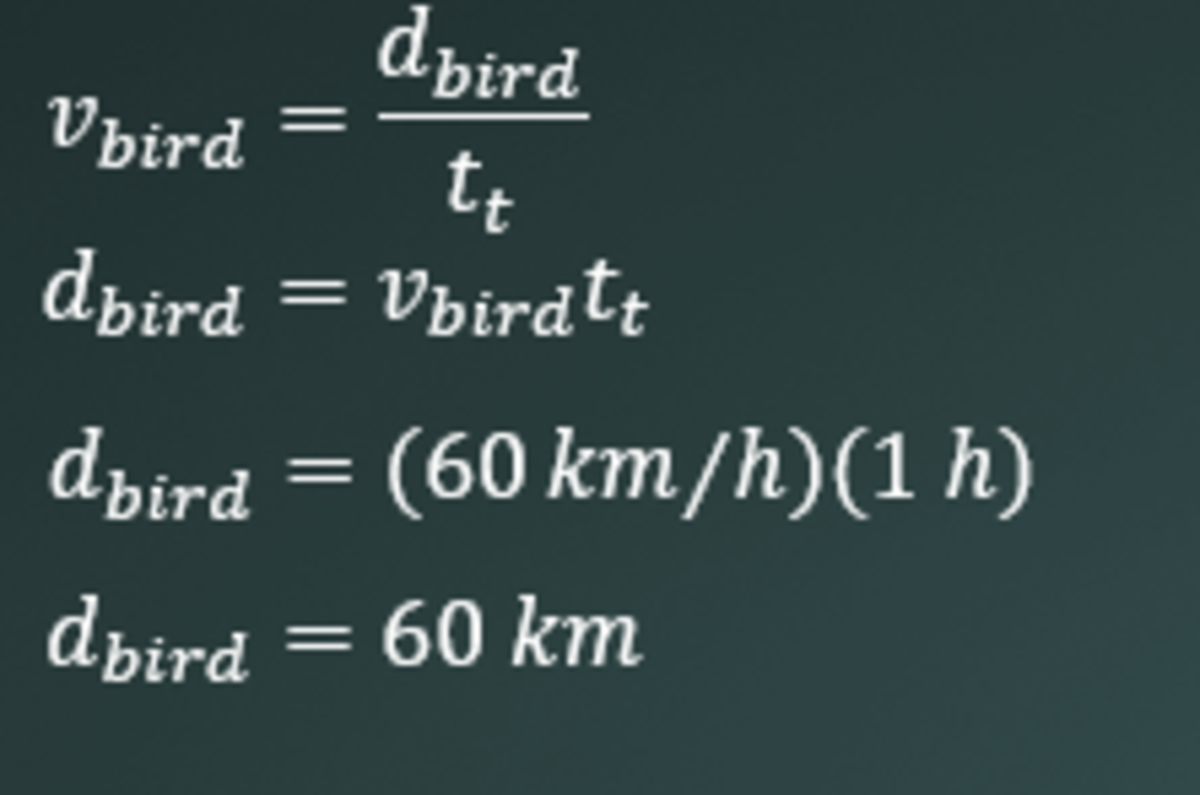3. Use All the Information You Have to Find the Distance of the Bird

You know that the bird only travels until the trains collide. You know that the trains collide in 1 hour. It's really simple. Use the velocity equation and sub in the time the trains take to collide in for the time. Solve for the bird's distance. Sub in your information and solve. (It was easier than you thought, I know!)

Answer: 60 km

## Comments

Lucas on September 12, 2013:

Cool problems post more!!!!